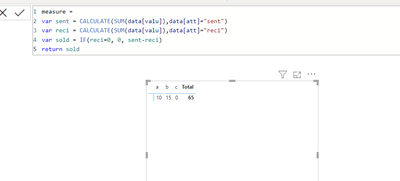cancel
Showing results for
Did you mean:New Member

## Subtracting from same column and then add up the differenceI have data kind of similar to this excel file in the PQ, I would like to get the difference of value column between sent and reci(att column) for each unique event ID

I was able to get that where for a the measure would be 10 and for b it would be 15 and for c 0 but the total is not adding up as it should be 25
I am trying to calculate it like thisCan some one help me with this,please?

1 ACCEPTED SOLUTIONSuper User

Hi @Varez42

=
SUMX ( 'Table', IF ( 'Table'[att] = "reci", - 'Table'[Value], 'Table'[Value] ) )

3 REPLIES 3Super User

Hi @Varez42

=
SUMX ( 'Table', IF ( 'Table'[att] = "reci", - 'Table'[Value], 'Table'[Value] ) )New Member

HI @KeyurPatel14
thanks for the response,
So based on the above data , I am trying something like this but as you can see the total should be 50 but it is not .Resolver IV

Hi @Varez42 ,
Can you please provide sample output for better understanding?